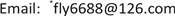1. 引言

Barker M和Rayens W  从理论上证明了源于计量化学领域回归问题的以解决多重共线性为目的偏最小二乘法与Fisher线性判别法之间的关系。本文采取了基于偏最小二乘(PLS)和主成分(PCA)的降维方法，分别对于与食管鳞癌相关的血清miRNAs数据降维处理，并进一步采用线性判别分析(LDA)，二次判别分析(QDA)，逻辑斯蒂判别(Log)，支持向量机(SVM)等判别方法，把数据分为训练集和测试集，利用十折交叉验证的方法，在测试集上得到了较高的分类准确率。

2. 数据与方法2.1. 数据来源

2.2. 方法

2.2.1. 基因选择

t = x ¯ 0 − x ¯ 1 s 0 2 N 0 + s 1 2 N 1

2.2.2. 降维方法

1) 偏最小二乘降维法 

arg max { [ cov ( a T x , b T y ) ] 2 ( a T a ) ( b T b ) } = { a } (1)

a为矩阵 Σ x y Σ y x 的最大的特征值所对应的特征向量。其中 Σ x y 为自变量与响应变量的协方差矩阵。把

a成为响应变量与自变量之间的最大偏最小二乘方向。

λ p ≤ x ′ B x x ′ x ≤ λ 1

arg max { [ cov ( a T x , b T y ) ] 2 ( a T a ) ( b T b ) } = arg max { ( a T Σ x y b ) 2 ( a T a ) ( b T b ) } = arg max { 1 ( a T a ) ( b T Σ y x a ) ( a T Σ x y b ) ( b T b ) } = arg max { a T Σ x y Σ y x a ( a T a ) }

a为矩阵 Σ x y Σ y x 的最大的特征值所对应的特征向量， b = Σ y x a 。

1) arg max { [ cov ( a h T X h − 1 , b h T Y h − 1 ) ] 2 ( a h T a h ) ( b h T b h ) } = a h ；

2) ξ h = X h − 1 a h ；

3) t h = X h − 1 T ξ h / ( ξ h T ξ h ) ；

4) X h = X h − 1 − ξ h t h T ；

5) X → ( ξ 1 , ξ 2 , ξ 3 ) 。

b) 主成分降维法 

arg max a ′ a = 1 [ cov ( a T x ) ] = a k

2.3. 判别方法

3. 结果及其分析

Comparisons of classification error rates of four discrimination methods under two dimension reduction methods. Classification results of serum microRNAs data in training set and test set. Each value in the table is error classification percentage averaged over 100 times of 10-fold cross validation. Perfect error classification is 0.167
mLogLDAQDASVM
PLSPCAPLSPCAPLSPCAPLSPCA
200.17730.27970.17440.27630.35460.28400.1685*0.2648#
0.13110.25990.12900.26000.12890.22740.1291*0.2586#
500.18030.25880.18870.25760.40910.30780.1675**0.2484##
0.12790.23540.14520.24320.14570.25630.1507**0.2293##

4. 讨论